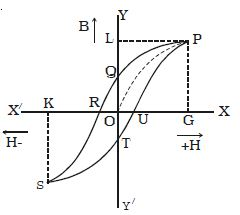# Describe on Hysteresis Loop in Magnetizing Field

Consider an iron bar being magnetized slowly by a magnetizing field H whose strength can be changed. It is found that the magnetic induction B inside the material increases with the strength of the magnetizing field and then attains a saturated level. This is depicted by the path OP in the Figure.If the magnetizing field is now decreased slowly, then magnetic induction also decreases but it does not follow the path PO. Instead, when H = 0, B has non zero value equal to OQ. This implies that some magnetism is left in the specimen. The value of magnetic induction of a substance, when the magnetizing field is reduced to zero, is called remanance or residual magnetic induction of the material. OQ represents the residual magnetism of the material. Now, if we apply the magnetizing field in the reverse direction, the magnetic induction decreases along QR till it becomes zero at R. Thus to reduce the residual magnetism (remanent magnetism) to zero, we have to apply a magnetizing field OR in the opposite direction.

The value of the magnetizing field H which has to be applied to the magnetic material in the reverse direction so as to reduce its residual magnetism to zero is called its coercivity.

When the strength of the magnetizing field H is further increased in the reverse direction, the magnetic induction increases along RS till it acquires saturation at a point S (points P and S are symmetrical). If we now again change the direction of the field, the magnetic induction follows the path STUD. This closed curve PQRSTUP is called the ‘hysteresis loop’ and it represents a cycle of magnetization. The word ‘hysteresis’ literally means lagging behind. We have seen that magnetic induction B lags behind the magnetizing field H in a cycle of magnetization. This phenomenon of lagging of magnetic induction behind the magnetizing field is called hysteresis.